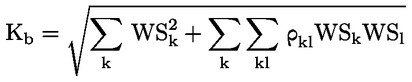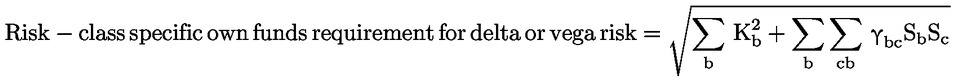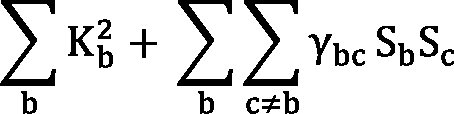In force

Version from: 01/01/2023
Amendments
Search within this legal act

### Article 325f - Own funds requirements for delta and vega risks

Article 325f

Own funds requirements for delta and vega risks

1.
Institutions shall apply the delta and vega risk factors described in Subsection 1 of Section 3 to calculate the own funds requirements for delta and vega risks.
2.
Institutions shall apply the process set out in paragraphs 3 to 8 to calculate own funds requirements for delta and vega risks.
3.
For each risk class, the sensitivity of all instruments in scope of the own funds requirements for delta or vega risks to each of the applicable delta or vega risk factors included in that risk class shall be calculated by using the corresponding formulas in Subsection 2 of Section 3. If the value of an instrument depends on several risk factors, the sensitivity shall be determined separately for each risk factor.
4.
Sensitivities shall be assigned to one of the buckets ‘b’ within each risk class.
5.
Within each bucket ‘b’, the positive and negative sensitivities to the same risk factor shall be netted, giving rise to net sensitivities (sk) to each risk factor k within a bucket.
6.

The net sensitivities to each risk factor within each bucket shall be multiplied by the corresponding risk weights set out in Section 6, giving rise to weighted sensitivities to each risk factor within that bucket in accordance with the following formula:

WSk = RWk · sk
where:
 WSk = the weighted sensitivities;
 RWk = the risk weights; and
 sk = the risk factor.
7.

The weighted sensitivities to the different risk factors within each bucket shall be aggregated in accordance with the formula below, where the quantity within the square root function is floored at zero, giving rise to the bucket-specific sensitivity. The corresponding correlations for weighted sensitivities within the same bucketkl), set out in Section 6, shall be used.where:
 Kb = the bucket-specific sensitivity; and
 WS = the weighted sensitivities.
8.

The bucket-specific sensitivity shall be calculated for each bucket within a risk class in accordance with paragraphs 5, 6 and 7. Once the bucket-specific sensitivity has been calculated for all buckets, weighted sensitivities to all risk factors across buckets shall be aggregated in accordance with the formula below, using the corresponding correlations γbc for weighted sensitivities in different buckets set out in Section 6, giving rise to the risk-class specific own funds requirement for delta or vega risk:where:

 Sb = Σk WSk for all risk factors in bucket b and Sc = Σk WSk in bucket c; where those values for Sb and Sc produce a negative number for the overall sum of, the institution shall calculate the risk-class specific own funds requirements for delta or vega risk using an alternative specification whereby
 Sb = max [min (Σk WSk, Kb), – Kb] for all risk factors in bucket b and
 Sc = max [min (Σk WSk, Kc), – Kc] for all risk factors in bucket c.

The risk-class specific own funds requirements for delta or vega risk shall be calculated for each risk class in accordance with paragraphs 1 to 8.